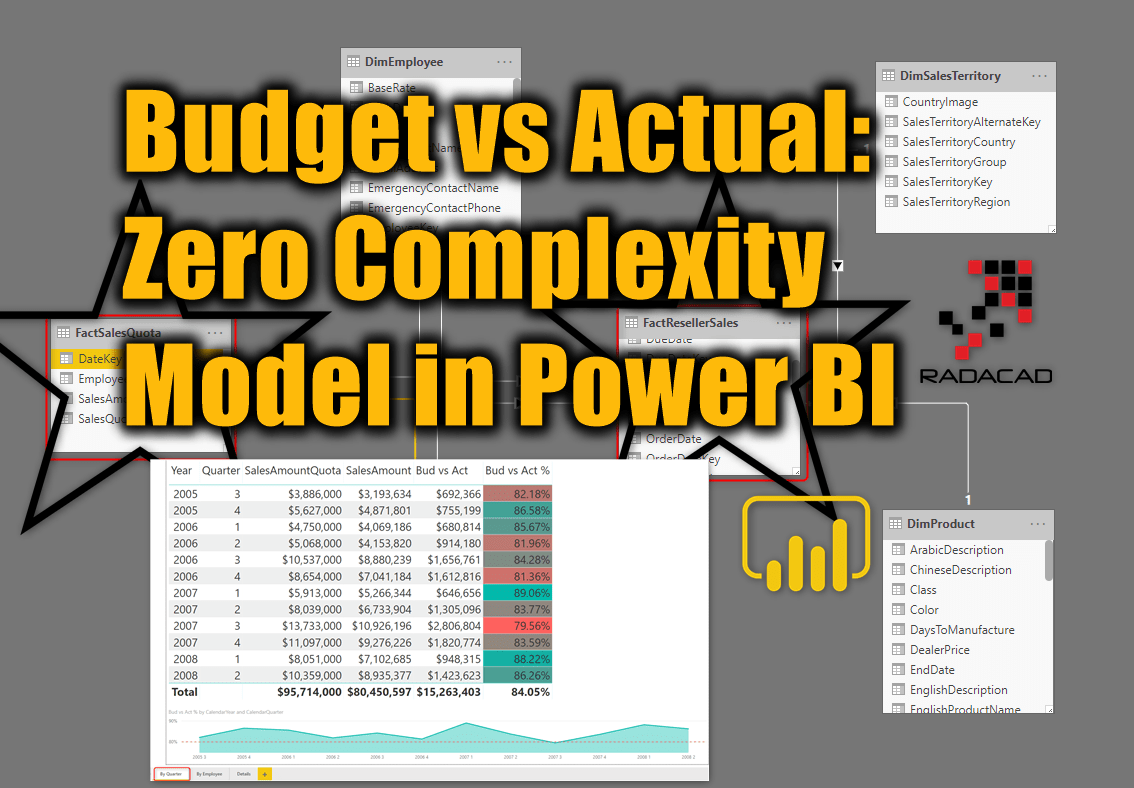# Budget vs. Actual Model in Power BI: Calculations and DAX

In the previous article, I explained how you can build a data model for budget vs. actual, where the grain of the two fact tables is different. The model works perfectly as a star schema. As long as you slice and dice data in the level of granularity that both tables support, then you don’t Read more about Budget vs. Actual Model in Power BI: Calculations and DAX[…]# Budget vs Actual: Zero Complexity Model in Power BI

When I explain the Star Schema and best practices of data modeling in Power BI, Often I get questions such as what if we want to have budget and actuals in the same data model? what if there are two tables with different granularity? We cannot solve it with simple data modeling and relationships, this Read more about Budget vs Actual: Zero Complexity Model in Power BI[…]